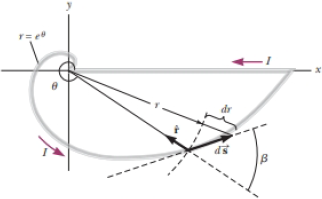Chapter 29, Problem 47CPPhysics for Scientists and Enginee...

10th Edition
Raymond A. Serway + 1 other
ISBN: 9781337553278

Solutions

Chapter
SectionPhysics for Scientists and Enginee...

10th Edition
Raymond A. Serway + 1 other
ISBN: 9781337553278
Textbook Problem

A wire carrying a current I is bent into the shape of an exponential spiral, r = eθ, from θ = 0 to θ = 2π as suggested in Figure P29.47. To complete a loop, the ends of the spiral are connected by a straight wire along the x axis. (a) The angle β between a radial line and its tangent line at any point on a curve r = f(θ) is related to the function by tan β = r d r / d θ Use this fact to show that β = π/4. (b) Find the magnetic field at the origin.Figure P29.47(a)

To determine

To show: The angle β between a radial line and its tangent line at any point on a curve r=f(θ) is π4 .

Explanation

Given info: The value of r=eθ from θ to 2π .

Formula to calculate the differentiation of the exponential spring is,

drdθ=d(eθ)dθ=eθ

Write the expression for the angle between a radial line and its tangent line is,

tanβ=rdr/dθ

Substitute

(b)

To determine
The magnetic field at origin.

Still sussing out bartleby?

Check out a sample textbook solution.

See a sample solution

The Solution to Your Study Problems

Bartleby provides explanations to thousands of textbook problems written by our experts, many with advanced degrees!

Get Started

Why do we use different map projections?

Fundamentals of Physical Geography

What is hereditarianism, and what is the invalid assumption it makes?

Human Heredity: Principles and Issues (MindTap Course List)

What is a hot spot? Where can you go to see hot-spot activity?

Oceanography: An Invitation To Marine Science, Loose-leaf Versin